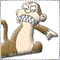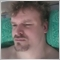# Indicator Calculation Loops.215

Could someone please explain how to ensure the indicator calculates all bars available on the first run, and then only the bar that most recently appears. My indicator calculates what I want on the first run, but then does nothing. I can't figure out why.

```if(_BarsCalculated==0) {
StartIndex = _BarsTotal-2;
FinishIndex = 1;
}
else {
StartIndex = 1;
FinishIndex = 1;
}

//TODO: Adjust arrow width depending on current chart zoom.
if(_BarsTotal<StartIndex) {
return(0);
}
else {
if(FirstRun) {

ArrayInitialize(HighCloseBullPattern,EMPTY_VALUE);
ArrayInitialize(MidCloseBullPattern,EMPTY_VALUE);
ArrayInitialize(LowCloseBullPattern,EMPTY_VALUE);
ArrayInitialize(HighCloseRangePattern,EMPTY_VALUE);
ArrayInitialize(MidCloseRangePattern,EMPTY_VALUE);
ArrayInitialize(LowCloseRangePattern,EMPTY_VALUE);
ArrayInitialize(HighCloseBearPattern,EMPTY_VALUE);
ArrayInitialize(MidCloseBearPattern,EMPTY_VALUE);
ArrayInitialize(LowCloseBearPattern,EMPTY_VALUE);

// Set the price arrays to match with the indicator arrays

FirstRun=false;
}
}

for(int Bar=StartIndex; Bar>=FinishIndex; Bar--) {
Sentiment = CandleSentiment(Bar,Bar+1,Bar1RangePercent,Bar2RangePercent,O,H,L,C);
}
```16552

whitebloodcell:

Could someone please explain how to ensure the indicator calculates all bars available on the first run, and then only the bar that most recently appears. My indicator calculates what I want on the first run, but then does nothing. I can't figure out why.

I recommend you read the documentation. This is good article on it. The loop you want is:

```int OnCalculate(const int rates_total,
const int prev_calculated,
const datetime &time[],
const double &open[],
const double &high[],
const double &low[],
const double &close[],
const long &tick_volume[],
const long &volume[],
{
//---
int limit;
if(prev_calculated==0)   //--- If it is the first call go through all bars
limit=0;
else
limit=rates_total-1;  //--- Else just check the most current bar that has not yet finished

for(int i=limit;i<rates_total-1;i++)
{
}
//--- return value of prev_calculated for next call
return(rates_total);```215

Candles:

I recommend you read the documentation. This is good article on it. The loop you want is:

Thanks for your help. I wanted to calculate from left to right, and thought I needed to decrement therefore, but I was confused in the way the bars are numbered.16552

whitebloodcell:
Thanks for your help. I wanted to calculate from left to right, and thought I needed to decrement therefore, but I was confused in the way the bars are numbered.
If you want to do it this way then you can use ArraySetAsSeries to change the indexing order.Documentation on MQL5: Array Functions / ArraySetAsSeries
• www.mql5.com
Array Functions / ArraySetAsSeries - Documentation on MQL5